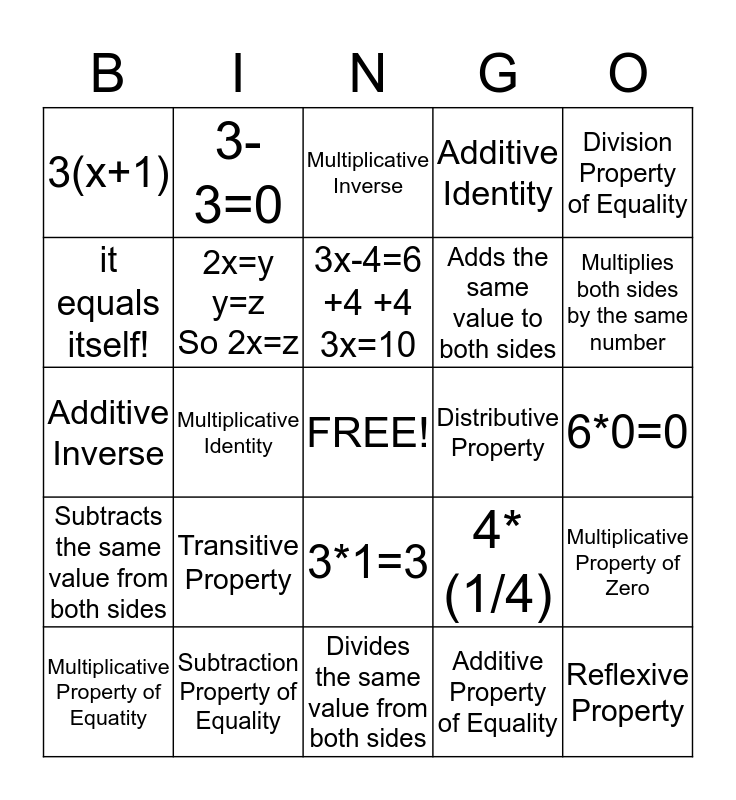# Algebra Properties Bingo!This bingo card has a free space and 24 words: Transitive Property, Subtraction Property of Equality, 3x-4=6 +4 +4 3x=10, Multiplicative Property of Equatity, Division Property of Equality, 3(x+1), it equals itself!, 3-3=0, Distributive Property, 3*1=3, Adds the same value to both sides, Additive Inverse, 4*(1/4), Multiplicative Property of Zero, 2x=y y=z So 2x=z, Additive Property of Equality, 6*0=0, Multiplicative Inverse, Multiplies both sides by the same number, Multiplicative Identity, Reflexive Property, Subtracts the same value from both sides, Additive Identity and Divides the same value from both sides.

## Play Online